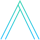# Kotlin Algorithm Challenge No. 2

December 24, 2019The following problem is the Longest Substring Without Repeating Characters problem on LeetCode.

Given a string, find the length of the longest substring without repeating characters.

Example 1:

.css-1baulvz{display:inline-block;}Input: "abcabcbb"Output: 3 Explanation: The answer is "abc", with the length of 3.

Example 2:

Input: "bbbbb"Output: 1Explanation: The answer is "b", with the length of 1.

Example 3:

Input: "pwwkew"Output: 3Explanation: The answer is "wke", with the length of 3.

There’s one nice example that comes up in the submissions that highlights an edge case as well, which is:

Input: "abba"Output: 2

# Solution

When we’re iterating through the array, and evaluating whether a new substring should be the max substring, we need to know two things:

• the length of the current max substring
• what characters are included in the current substring

Once we have those two things, it’s just a matter of iterating through the array once, and it’s not so complicated.

Getting the current max is simple, it’s just a matter of maintaining a max, initialized at 0, and update it as we go through the array.

## Getting the current substring

This part is a little bit more complicated.

abcabcbb^  ^i  j

Say we’re iterating through the above string. We’re at position j. The current substring we’re looking at is everything up until i.

If we can easily find i, then the length of the current substring is simply $j - i$. But how do we find i?

Speaking generally, i is the last occurrence of any char in our current substring.

We can break that into two cases:

1. i is the index of the last occurrence of the char we are currently sitting at, as we iterate through.

2. i is the index of the last occurrence of any other char in the current substring

I’ve renamed i to lastRepeat, because what it actually represents is the last repeat of any char in the current substring.

Here is the final code:

fun maxSubstring(str: String): Int {    val map: HashMap<Char, Int> = hashMapOf()    var max = 0    var lastRepeat = -1    str.forEachIndexed { j, value ->        val lastOccurence = map.get(value)        if (lastOccurence != null) {            lastRepeat = maxOf(lastRepeat, lastOccurence)        }                                max = maxOf(max, j - lastRepeat)        map.put(value, j)    }        return max}

# Complexity

We’re iterating through the array once, so the time complexity is $O(n)$.

The space usage is constant, because while we do have a hashmap, the maximum number of keys is limited by the total number of ASCII characters.

Here we’re the results of my submission on LeetCode.I was a bit curious why it wasn’t a bit higher in terms of time, given that you really can’t make it any more efficient in terms of iteration.

Turns out, forEachIndexed is less efficient than a simple for loop, e.g.

import kotlin.system.measureNanoTimeval x = (1..10000).toList().toTypedArray()fun foo(): Unit {}measureNanoTime { x.forEachIndexed { _, _ -> foo() } } // 674349measureNanoTime { for (temp in 0 until x.size) { foo() } } // 192791

Note that we’re talking in nanoseconds here—I definitely think the readability gains from Kotlin’s functional APIs are worth a few extra nanoseconds. But if you do care about that kind of thing:

fun maxSubstringWithForLoop(str: String): Int {    val map: HashMap<Char, Int> = hashMapOf()    var max = 0    var lastRepeat = -1        for (j in 0 until str.length) {        val value = str[j]                val lastOccurence: Int? = map.get(value)        if (lastOccurence != null) {            lastRepeat = maxOf(lastRepeat, lastOccurence)        }                                max = maxOf(max, j - lastRepeat)        map.put(value, j)                    }        return max}

And it’s a tiny bit faster.# Join the mailing list

Get the latest posts in your inbox. No spam, ever.